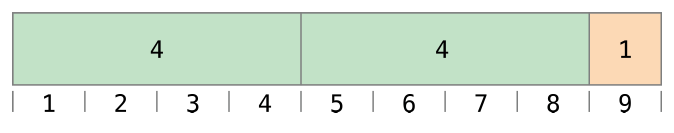# JavaScript: Loops & Arrays

Notes Home

## Arrays

An `array` is an object that contains a list of values or variables:

``````var imgs = ["cat.jpg", "dog.jpg", "bird.jpg"];
var decades = [1980, 1990, 2000, 2010, 2020];``````

In JavaScript, the items in an array can have any datatype, like a number, string, boolean, or even another arrary. In other languages, the data in an array usually are all of the same type. The elements of an array are accessed by their index number, starting with ``.

`imgs` will return `"cat.jpg"`. Like strings, arrays have a `length` property. Try creating an array, reading from the list, and returning the length:

``````var myArray = ["cat", "dog", "alligator"];
myArray.length;
myArray;
myArray;``````

## Adding elements to an array

``````var imgs = ["cat", "dog", "bird"];
imgs = "alligator";
imgs.push("lion");
imgs.pop();``````

Arrays have methods and properties that help us manipulate them.

A method is a function that is associated with an object.

A property is a variable value associated with an object.

``````var a = ["cat", "dog", "alligator"];
console.log(a.length);
var i = a.indexOf("dog");
console.log(i);``````

`.length` is a property, because it just stores one value, the number of elements in the array.

`.indexOf()` is a method, because, like a function, it takes an argument and runs a block of code which returns a value.

``console.log(a.indexOf);``

## Loops

A loop defines a set of commands to execute a certain number of times. JavaScript uses several loop statements, including `do`, `while` and `for`.

Loops look like other control statements, such as `if () { ... }` but run the code block a certain number of times.

A `while` statements executes as long as the first statement is true.

This is essentially like copying and pasting the same piece of code five times, while changing a number or input. Loops are an efficient way to excecute actions on a list or array of data.

``````var i = 0;
if (i < 5) {
i++;
console.log(i);
}``````
``````var i = 0;
while (i < 5) {
i++;
console.log(i);
}``````

`for` loops are more common in JavaScript, because they are a little safer to write. If you forget an increment statement in your while loop, you will create an infinite loop, which will cause a stack overflow and crash your browser window.

``````for (var i = 0; i < 5; i++) {
console.log(i);
}``````

The `for` keyword indicates the beginning of the for loop. Inside the parentheses there are three statements that control the duration of the loop.

The first statement establishes the initial condition: `var i = 0;`.

The second statement establishes a conditional: `i < 5;`. As long as this condition evaluates to `true`, the loop will continue.

The final statement is the incremental/decremental statement or iteration: `i++;`.

The code block for the loop is then contained in the curly brackets: `{ ... }`.

``````for (initialization; conditional; iteration;) {
loop body;
}``````

In many cases the initial condition will be the value `0` as in `var i = 0;` ("i" in this case is shorthand for index).

But our initial condition could be anything.

``````for (var i = 1; i < 6; i++) {
console.log(i);
}``````
``````for (var i = 1, len = i + 5; i < len; i++) {
console.log(i);
}``````
``````for (var i = 1, len = i + 5; i < len; i++) {
console.log(i);
}``````

Likewise, we can change the conditional and iteration statements.

``````for (var i = 0; i < 100; i += 10) {
console.log(i);
}``````
``````for (var i = 10; i > 0; i--) {
console.log(i);
}``````

A practical example of a loop in JavaScript:

`````` var imgs = ["cat.jpg", "dog.jpg", "bird.jpg"];
for (var i = 0, len = imgs.length; i < len; i++) {
console.log('<img src="'+imgs[i]+'">');
}``````

Note on scope: variables declared inside a `for` loop (or any other loop) are not scoped to the loop. Loops do not have the same scope priveleges of functions.

``````for (var i = 0; i < 5; i++) {
var x = i;
console.log(x);
}
console.log(x);``````

## Random

Like the array object, JavaScript also has a Math global object, which contains basic methods for manipulating numbers.

We can use the Math object to generate random numbers.

`Math.random()` generates a random number between 0 and 1.

``````var n = Math.random();
console.log(n);
a[n];
a;``````

Floating point numbers are not very useful for getting an element from an array, which indexes by ints.

We can use another Math method, `Math.floor()` to round the number down:

``````var n = Math.floor(Math.Random());
console.log(n);
a[n];``````

We need to adjust the range of our random values. Instead of a number between 0 and 1, we want a number between 0 (the first index of the array) and the last index of the array, or `array.length`

``````var n = Math.floor(Math.random() * a.length));
console.log(n);
a[n];``````

This is a common pattern in JavaScript.

``````var a = ["cat", "dog", "alligator"];
a[Math.floor(Math.random() * a.length)];``````

## Modulo

JavaScript has a mathematical operator called modulo `%` which gives the remainder of a division of two values.``````9 % 4;
5 % 4;
2 % 1;
1 % 2;
30 % 3;
31 % 3;
for (var i = 0; i < 20; i++) {
console.log(i % 5);
}``````

Notice that when we count up using modulo, we repeat the same count, even though `i` keeps going up.

This is useful for counting or flipping aspects of your program.